# 感知机

PLA 全称是 Perceptron Linear Algorithm，即线性感知机算法，属于一种最简单的感知机（Perceptron）模型。

## 核心思想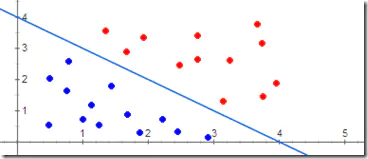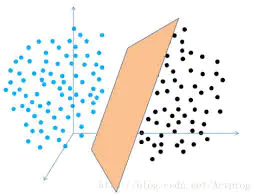### 不可分的场景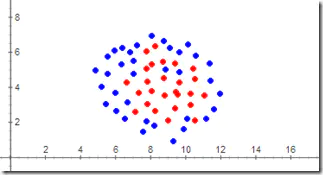# 基本结构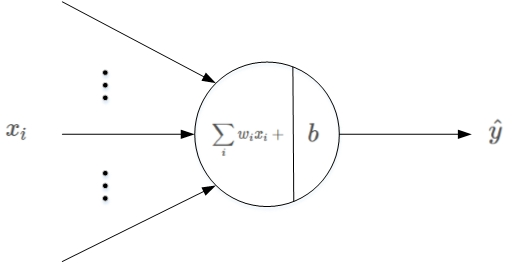## 线性输出

scores=∑i~N wi*xi+b


scores=∑i~N+1 wi*xi


## 阈值比较

scores 是感知机的输出，接下来就要对 scores 进行判断：

（1）若 scores≥0 ，则y^=1（正类）

（2）若 scores<0，则y^=−1（负类）

# PLA 理论解释

## 感知机算法的原始形式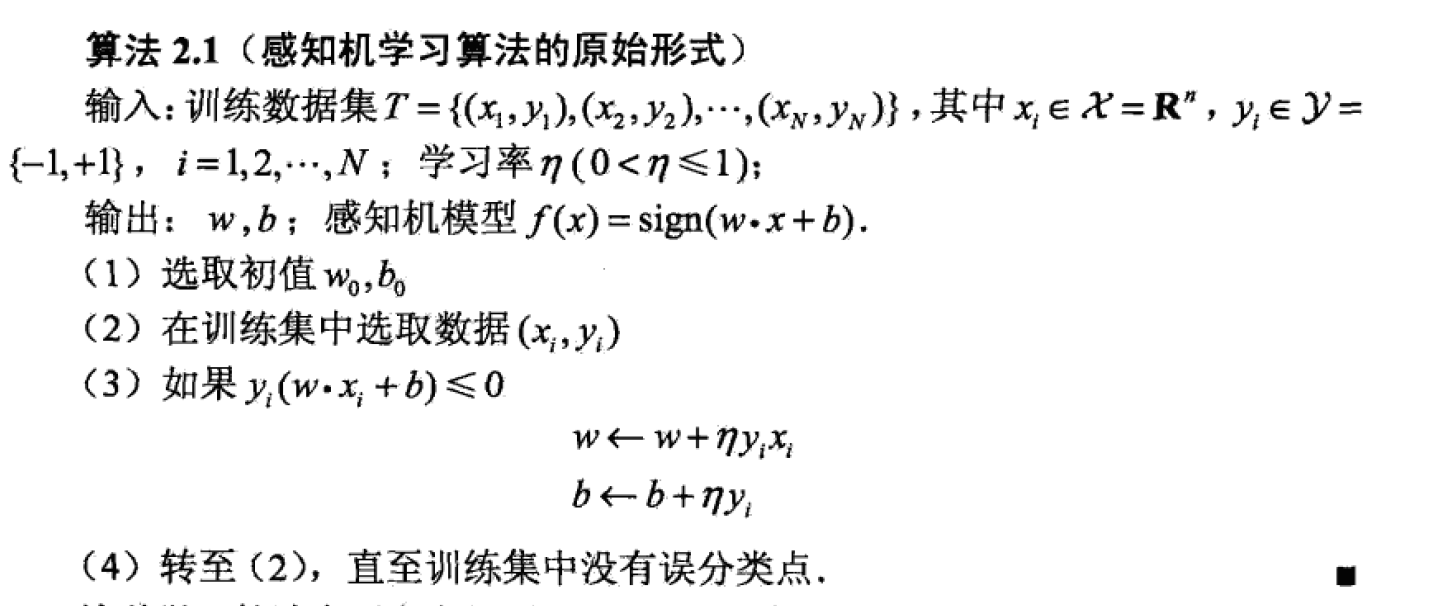## 理论解释

PLA的基本原理就是逐点修正，首先在超平面上随意取一条分类面，统计分类错误的点；然后随机对某个错误点就行修正，即变换直线的位置，使该错误点得以修正；接着再随机选择一个错误点进行纠正，分类面不断变化，直到所有的点都完全分类正确了，就得到了最佳的分类面。

## 例子

w:=w+x=w+yx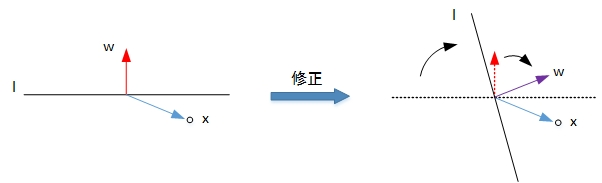w:=w−x=w+yx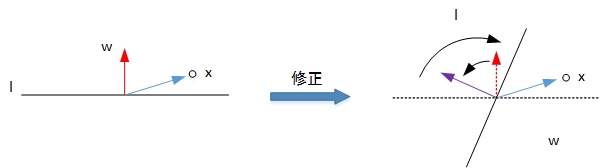# 算法实现

## 数据准备

https://gitee.com/houbinbin/ai-data/blob/master/pla/data/data1.csv

...
5.1,3,1
5.7,4.1,1
5.1,1.4,-1
4.9,1.4,-1
...


## 数据导入

import numpy as np
import pandas as pd

# 样本输入，维度（100，2）
x = data.iloc[:,:2].values
# 样本输出，维度（100，）
y = data.iloc[:,2].values


D:\\_data\\ai-data\\pla\\data1.csv 是这个 csv 在我本地的路径。

x 对应的就是前面的两个维度值：

array([[7. , 4.7],
[6.4, 4.5],
...
[4.8, 1.4],
[5.1, 1.6],
[4.6, 1.4],
[5.3, 1.5],
[5. , 1.4]])


y 对应的是最后的分类值：

array([ 1,  1,  1,  1,  1,  1,  1,  1,  1,  1,  1,  1,  1,  1,  1,  1,  1,
...
-1, -1, -1, -1, -1, -1, -1, -1, -1, -1, -1, -1, -1, -1, -1],
dtype=int64)


## 数据分类与可视化

import matplotlib.pyplot as plt

# 可视化
plt.scatter(x[:50, 0], x[:50, 1], color='blue', marker='o', label='Positive')
plt.scatter(x[50:, 0], x[50:, 1], color='red', marker='x', label='Negative')
plt.xlabel('Feature 1')
plt.ylabel('Feature 2')
plt.legend(loc = 'upper left')
plt.title('Original Data')
plt.show()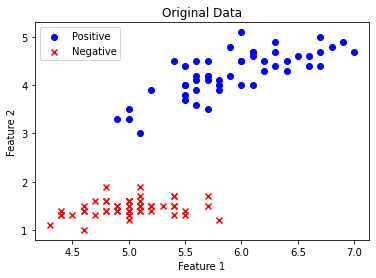## 特征归一化（normalization）介绍

### 归一化的方法

（1）线性比例变换

yi = xi / max(x)


（2）极差变换法

yi = (xi - min(x)) / (max(x)-min(x))


（3）Z-score standardization

yi = (xi - μ) / σ


## 数据归一化

# 归一化
# 均值
u = np.mean(x, axis=0)
# 方差
v = np.std(x, axis=0)
x = (x - u) / v

# 作图
plt.scatter(x[:50, 0], x[:50, 1], color='blue', marker='o', label='Positive')
plt.scatter(x[50:, 0], x[50:, 1], color='red', marker='x', label='Negative')
plt.xlabel('Feature 1')
plt.ylabel('Feature 2')
plt.legend(loc = 'upper left')
plt.title('Normalization data')
plt.show()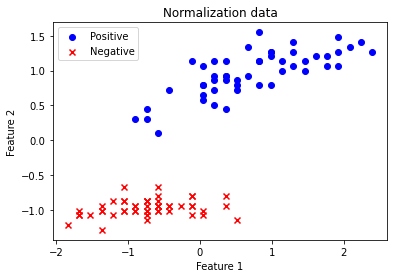## 直线初始化

# 初始化直线
# x加上偏置项
x = np.hstack((np.ones((x.shape,1)), x))
# 权重初始化
w = np.random.randn(3,1)

# 直线第一个坐标（x1，y1）
x1 = -2
y1 = -1 / w * (w * 1 + w * x1)
# 直线第二个坐标（x2，y2）
x2 = 2
y2 = -1 / w * (w * 1 + w * x2)
plt.plot([x1,x2], [y1,y2],'r')

plt.show()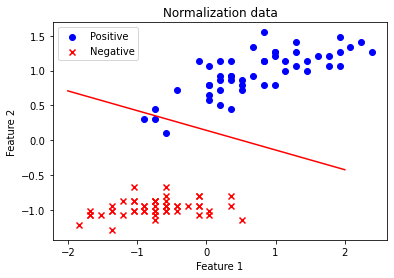### numpy 方法补充

• shape

shape 函数是numpy.core.fromnumeric中的函数，它的功能是查看矩阵或者数组的维数。

x.shape 是 100 维度。

• ones

np.ones((x.shape,1) 实际上返回的是一个 100 个元素都是 1 的数组。

• hstack

hstack表示轴1合并。

hstack的字母h来自于horizontal，表示两个数组是水平的，hstack((a,b))将把b排在a的右边的意思。

print(np.hstack([[1,2],]))
# [1,2,3]


• random.randn

numpy.random.randn(d0,d1,…,dn)

randn函数返回一个或一组样本，具有标准正态分布。dn表格每个维度。

array([[0.64171487],
[0.74705545],
[0.66654185]])


## 计算 scores

s = np.dot(x, w)
y_pred = np.ones_like(y)      # 预测输出初始化
loc_n = np.where(s < 0)    # 小于零索引下标
y_pred[loc_n] = -1


# 第一个分类错误的点
t = np.where(y != y_pred)
# 更新权重w
w += y[t] * x[t, :].reshape((3,1))


### 数组方法简介

x[t, :] 表示裁剪数组，从 t 到最后。

x[0,:].reshape((3,1)) 就是把 3*1 转换为 3 行，1列的矩阵：

array([[1.        ],
[2.39474331],
[1.27506221]])


y * x[0,:].reshape((3,1))
=
array([[1.        ],
[2.39474331],
[1.27506221]])


### numpy 方法说明

• dot

np.dot(x, w) 实际上就是矩阵 x 与矩阵 w 的乘积。

• ones_like

• where(condition)

loc_n = np.where(s < 0)    # 小于零索引下标
y_pred[loc_n] = -1  # 把矩阵乘积 wi*xi 小于0 的位置，设置为 -1


## 迭代更新训练

（注意，前提是正负样本完全可分）

for i in range(100):
s = np.dot(x, w)
y_pred = np.ones_like(y)
loc_n = np.where(s < 0)
y_pred[loc_n] = -1

num_fault = len(np.where(y != y_pred))
print('第%2d次更新，分类错误的点个数：%2d' % (i, num_fault))
if num_fault == 0:
break
else:
t = np.where(y != y_pred)
w += y[t] * x[t, :].reshape((3,1))


# 直线第一个坐标（x1，y1）
x1 = -2
y1 = -1 / w * (w * 1 + w * x1)
# 直线第二个坐标（x2，y2）
x2 = 2
y2 = -1 / w * (w * 1 + w * x2)
# 作图
plt.scatter(x[:50, 1], x[:50, 2], color='blue', marker='o', label='Positive')
plt.scatter(x[50:, 1], x[50:, 2], color='red', marker='x', label='Negative')
plt.plot([x1,x2], [y1,y2],'r')
plt.xlabel('Feature 1')
plt.ylabel('Feature 2')
plt.legend(loc = 'upper left')
plt.show()


### x,y 的问题

# 直线第一个坐标（x1，y1）
x1 = -2
y1 = -1 / w * (w * 1 + w * x1)
# 直线第二个坐标（x2，y2）
x2 = 2
y2 = -1 / w * (w * 1 + w * x2)


w*1 + w*x1 + w*y1=0


## 完整的代码

• PLA.py
# PLA算法
import numpy as np
import pandas as pd
import matplotlib.pyplot as plt

# 数据读取
# 样本输入，维度（100，2）
x = data.iloc[:,:2].values
# 样本输出，维度（100，）
y = data.iloc[:,2].values

# 数据归一化
u = np.mean(x, axis=0)
v = np.std(x, axis=0)
x = (x - u) / v

# 直线初始化
# x加上偏置项
x = np.hstack((np.ones((x.shape,1)), x))
# 权重初始化
w = np.random.randn(3,1)

# 迭代更新
for i in range(100):
s = np.dot(x, w)
y_pred = np.ones_like(y)
loc_n = np.where(s < 0)
y_pred[loc_n] = -1
num_fault = len(np.where(y != y_pred))
print('第%2d次更新，分类错误的点个数：%2d' % (i, num_fault))
if num_fault == 0:
break
else:
t = np.where(y != y_pred)
w += y[t] * x[t, :].reshape((3,1))

# 直线第一个坐标（x1，y1）
x1 = -2
y1 = -1 / w * (w * 1 + w * x1)
# 直线第二个坐标（x2，y2）
x2 = 2
y2 = -1 / w * (w * 1 + w * x2)
# 作图
plt.scatter(x[:50, 1], x[:50, 2], color='blue', marker='o', label='Positive')
plt.scatter(x[50:, 1], x[50:, 2], color='red', marker='x', label='Negative')
plt.plot([x1,x2], [y1,y2],'r')
plt.xlabel('Feature 1')
plt.ylabel('Feature 2')
plt.legend(loc = 'upper left')
plt.show()


第 0次更新，分类错误的点个数： 3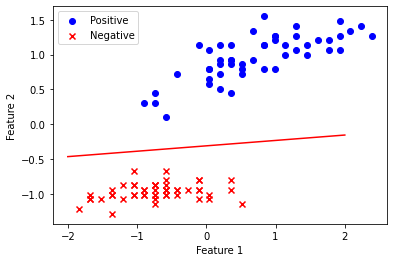《统计学习方法》